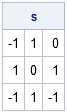The other day I needed to compute the signum function for each element of a matrix. If x is a real number, then the sgn(x) is -1 when x<0, 1 when x>0, and 0 when x=0.

I wrote a SAS/IML module that contains a compact little expression:

```proc iml; start sgn(x); return( (x>=0) - (x<=0) ); finish;```

However, even before I tested the function, I heard a little voice inside my head calling "I'll bet SAS already has this function...." A short search later, and I had discovered the built-in SIGN function:

```x = {-3 2 0, 5 0 7, -2 1 -5 }; s = sign(x); print s;```Lessons learned:

Share